# simulink Problem 3: Create a Simulink model to plot the solution of the following equation for...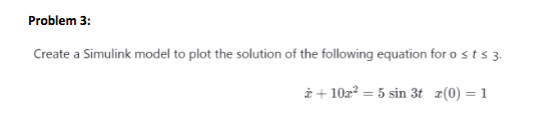Problem 3: Create a Simulink model to plot the solution of the following equation for osts 3. +10x2 = 5 sin 3t x(0) = 1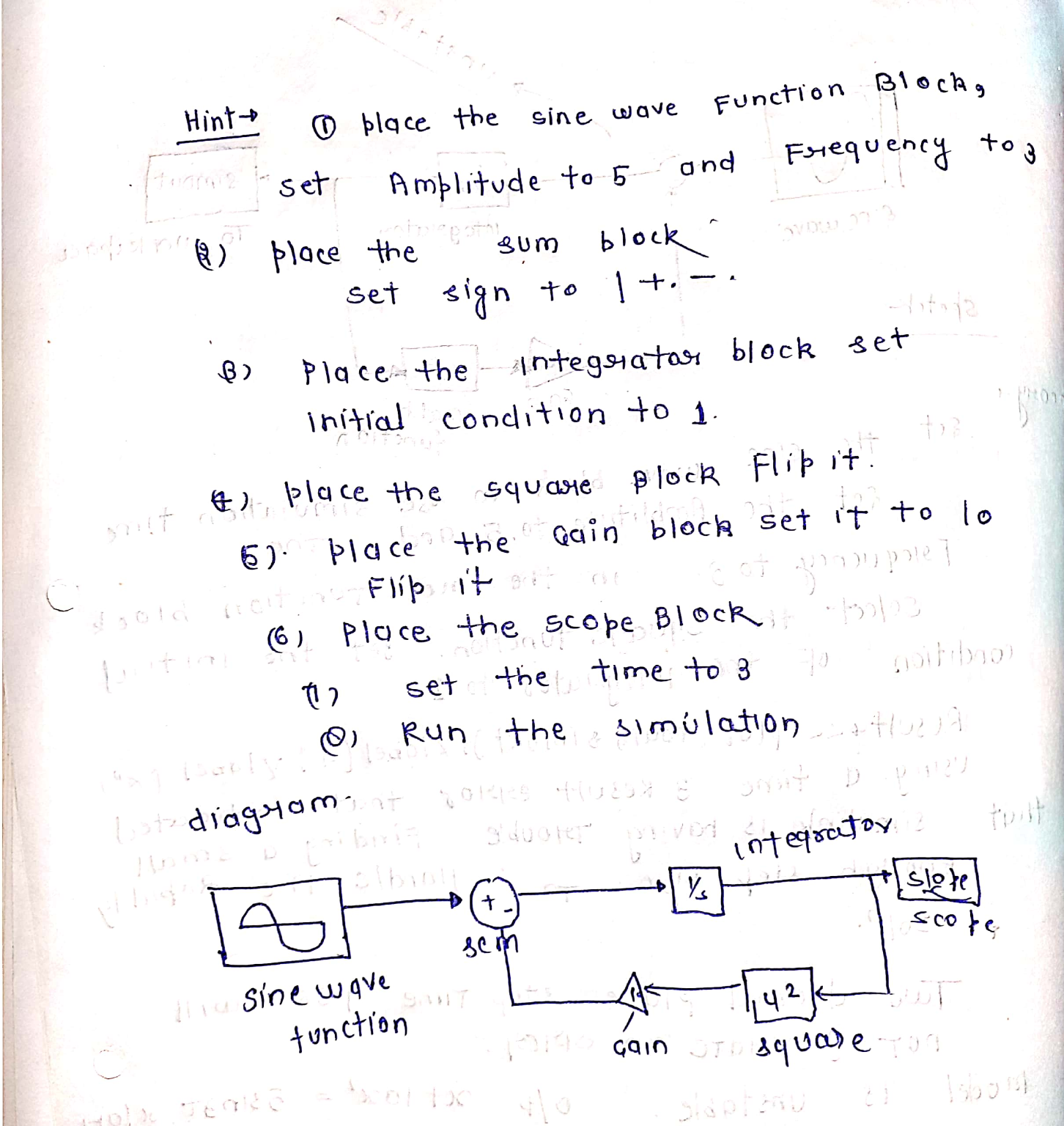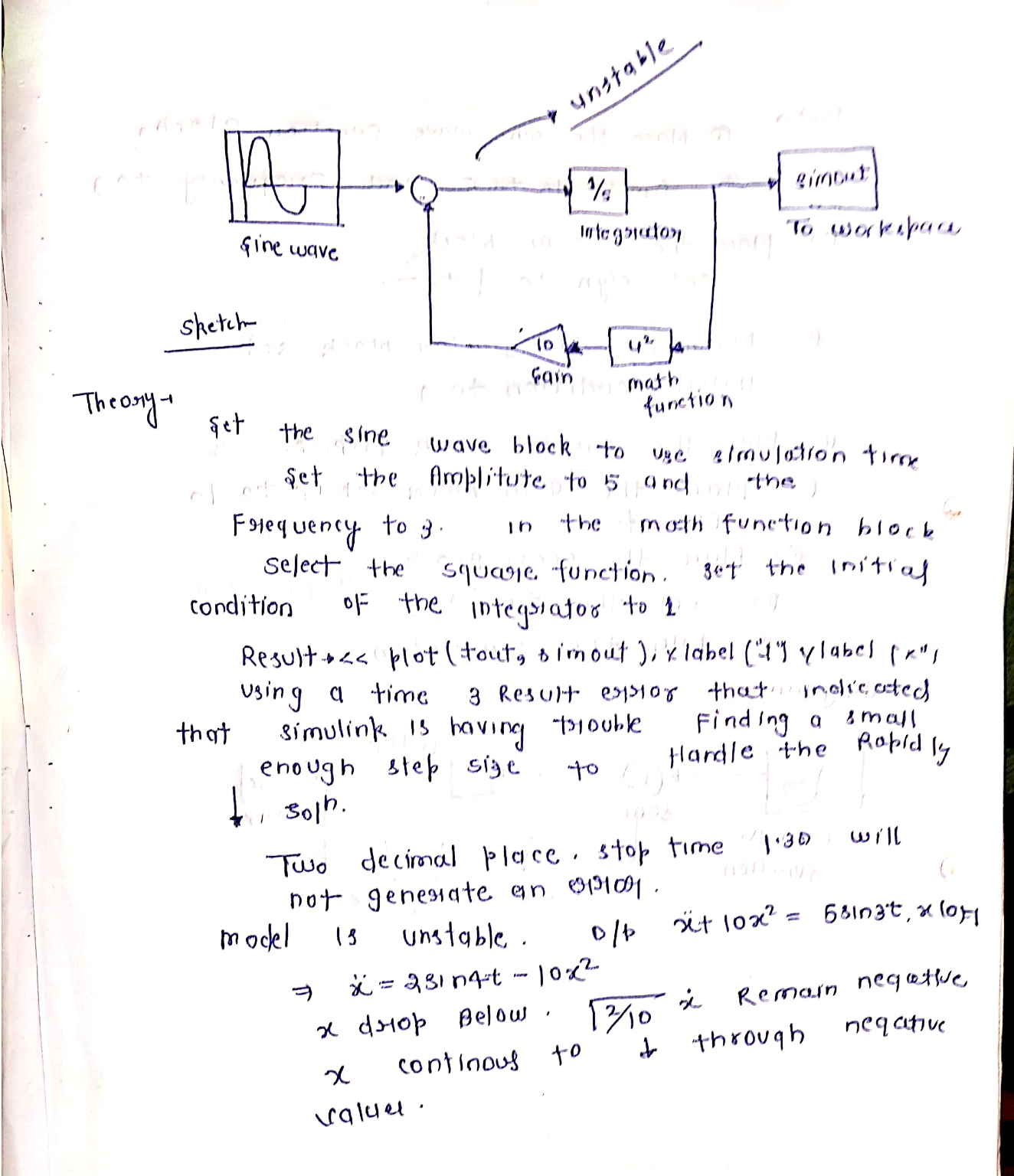Similar Homework Help Questions

Use the saturation block to create a Simulink model to plot the solution of the following equation for 0<=t<=63y' + y = f(t)where f(t) = 8 if 10sin(3t) > 8f(t) = -8 if 10sin(3t) < -8f(t) = 10sin(3t) otherwise(Peice-wise defined funtion)Will Rate Lifesaver+++++Thanks in advance

• ### Construct a Simulink model of the following problem 5 xdot + sin x = f (t), x(0) = 0; f(t) = -...

Construct a Simulink model of the following problem 5 xdot + sin x = f (t), x(0) = 0; f(t) = -5 if ψ(t) <= -5 ψ(t) if -5<=ψ(t)<=5 5 if ψ(t)>=5 where ψ(t) = 10 sin 4t

• ### Using MATLAB_R2017a, solve #3 using the differential equation in question #2 using Simulink, pres...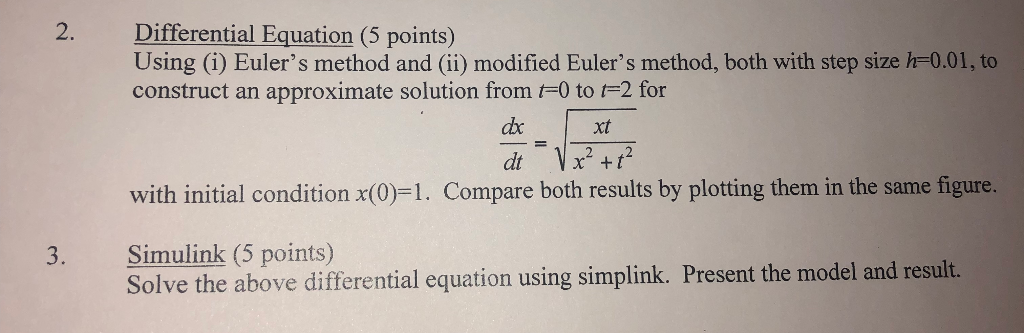Using MATLAB_R2017a, solve #3 using the differential equation in question #2 using Simulink, present the model and result. 2. Differential Equation (5 points) Using (i) Euler's method and (ii) modified Euler's method, both with step size h-0.01, to construct an approximate solution from F0 to F2 for xt 2, 42 with initial condition x(0)=1. Compare both results by plotting them in the same figure. 3. Simulink (5 points) Solve the above differential equation using simplink. Present the model and result....

• ### A colleague gave you a Simulink model (figure 5) for a non-linear mass-spring-damper system but f...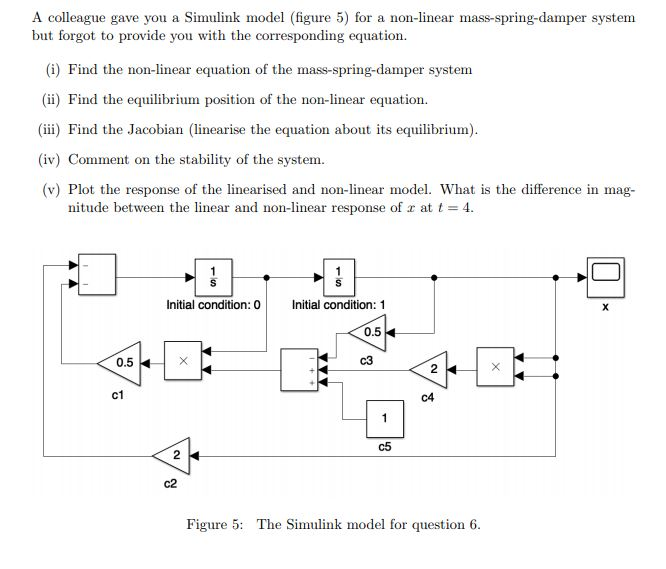A colleague gave you a Simulink model (figure 5) for a non-linear mass-spring-damper system but forgot to provide yo u with the corresponding equation. (i) Find the non-linear equation of the mass-spring-damper system (ii) Find the equilibrium position (iii Find the Jacobian (linearise the equation about ts equilibrium) (iv) Comment on the stability of the system. (v) Plot the response of the linearised and non-linear model. What is the difference in mag of the non-linear equation. nitude between the linear...

• ### please solve -Mathematical model -Mathlab Simulink digram -Plot diagram thank you 6.10 A series RL circuit with a noalinear inductor is shown in Fig. P6.10. Recall that the following nonlinear functi...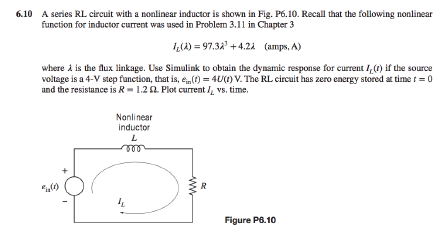please solve -Mathematical model -Mathlab Simulink digram -Plot diagram thank you 6.10 A series RL circuit with a noalinear inductor is shown in Fig. P6.10. Recall that the following nonlinear function for inductor current was used in Problem 3.11 in Chapter 3 4(A) = 97.32 +42a (amps, A) where a is the aux linkage. Use Simulink to obtain the dynamic response for current ILU) ifthe source voltage is a 4-V step function, that is, ein(t) = 4U(1) V. The RL...

• ### 5. Exporting your filter designs in 4(a) and 4(b) to SIMULINK In SIMULINK, create two discrete-time signals x1n],x2...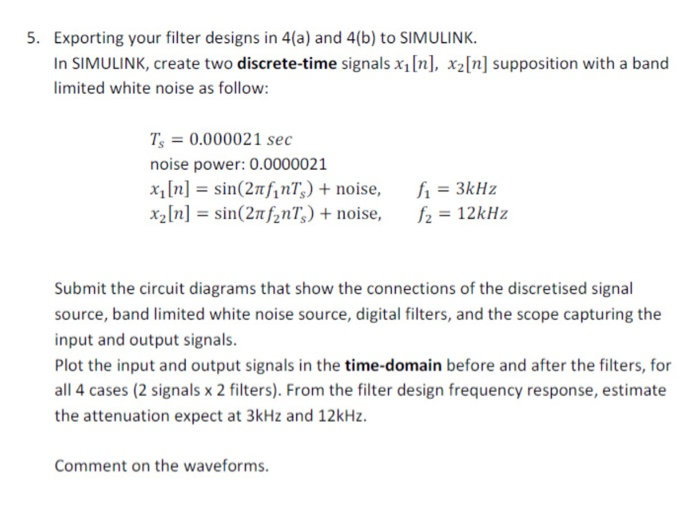5. Exporting your filter designs in 4(a) and 4(b) to SIMULINK In SIMULINK, create two discrete-time signals x1n],x2In] supposition with a band limited white noise as follow T, = 0.000021 sec noise power: 0.0000021 x1 [n] = sin(2rhn%) + noise, X2[n] = sin(2thn7,) + noise, f, = 3kHz f, = 12kHz Submit the circuit diagrams that show the connections of the discretised signal source, band limited white noise source, digital filters, and the scope capturing the input and output signals...

• ### PROBLEM 1 IS SUPPOSED TO BE A WAVE EQUATION NOT HEAT EQUATION 1. Find the solution...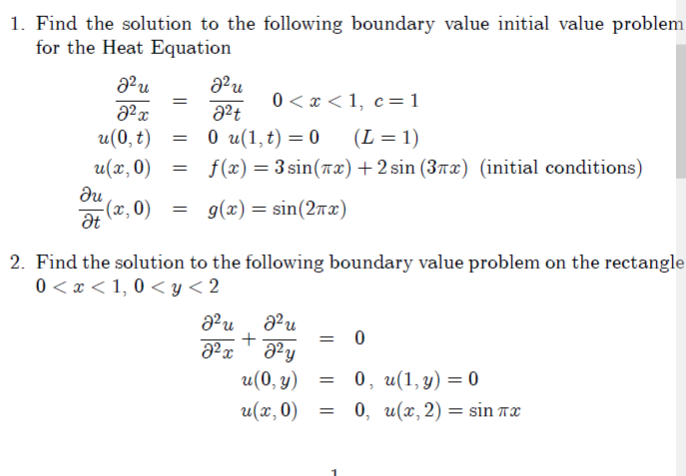PROBLEM 1 IS SUPPOSED TO BE A WAVE EQUATION NOT HEAT EQUATION 1. Find the solution to the following boundary value initial value problem for the Heat Equation au 22u 22 = 22+ 2 0<x<1, c=1 <3 <1, C u(0,t) = 0 u(1,t) = 0 (L = 1) u(x,0) = f(x) = 3 sin(7x) + 2 sin (3x) (initial conditions) (2,0) = g(x) = sin(2x) 2. Find the solution to the following boundary value problem on the rectangle 0 <...

• ### Build up a SIMULINK model of the engine-propeller system with the following governing equations o...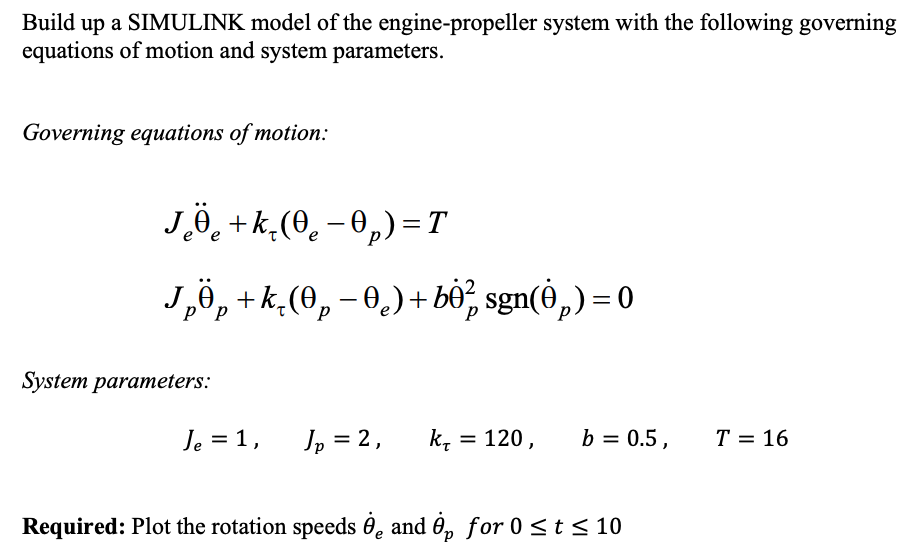Build up a SIMULINK model of the engine-propeller system with the following governing equations of motion and system parameters Governing equations of motion J,9, + k.(9, _ θ.) + bé, [email protected])-0 System parameters: Je1 Jp-2, kr-120, b-0.5, T-16 Required: Plot the rotation speeds θ, and ëp for 0 10 Build up a SIMULINK model of the engine-propeller system with the following governing equations of motion and system parameters Governing equations of motion J,9, + k.(9, _ θ.) + bé, [email protected])-0...

• ### a) Given Model if-else control flow in Matlab Simulink, explain the following result Scope Subsystemt Sine Wave up 200 150 50 100 Constantt Time offset 0 3t2 + 2t -0.5 b) Sketch model to plot the...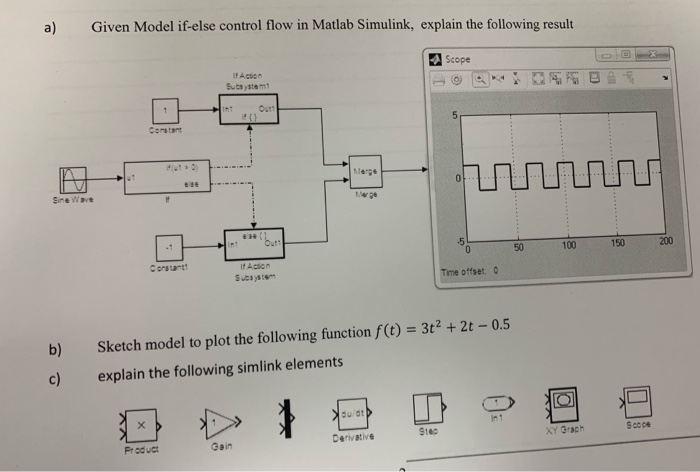a) Given Model if-else control flow in Matlab Simulink, explain the following result Scope Subsystemt Sine Wave up 200 150 50 100 Constantt Time offset 0 3t2 + 2t -0.5 b) Sketch model to plot the following function f(t) c) explain the following simlink elements du/dt Scoce Stec Derivative Gain Produc a) Given Model if-else control flow in Matlab Simulink, explain the following result Scope Subsystemt Sine Wave up 200 150 50 100 Constantt Time offset 0 3t2 + 2t...

• ### Sketch the Simulink model to model: x = 3+5 *t+6/(t+1 ) y = t^2-1/(2+t) where t is the time in se...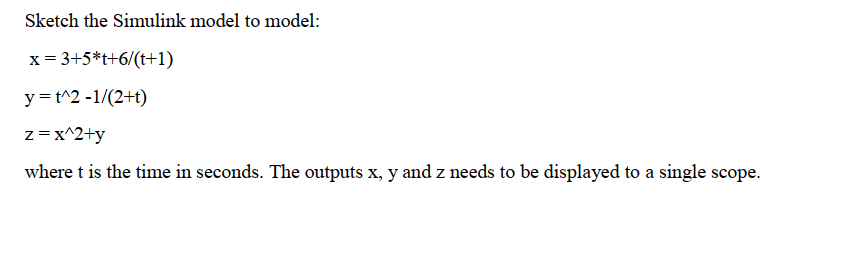Sketch the Simulink model to model: x = 3+5 *t+6/(t+1 ) y = t^2-1/(2+t) where t is the time in seconds. The outputs x, y and z needs to be displayed to a single scope. Sketch the Simulink model to model: x = 3+5 *t+6/(t+1 ) y = t^2-1/(2+t) where t is the time in seconds. The outputs x, y and z needs to be displayed to a single scope.

Free Homework App